Next: Exercises (N.B. Neglect spin Up: Multi-Particle Systems Previous: Two-Particle Systems

# Identical Particles

Consider a system consisting of two identical particles of mass. As before, the instantaneous state of the system is specified by the complex wavefunction. However, the only thing which this wavefunction tells us is that the probability of finding the first particle between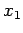and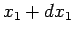, and the second between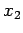and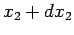, at timeis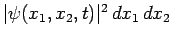. However, since the particles are identical, this must be the same as the probability of finding the first particle betweenand, and the second betweenand, at time(since, in both cases, the physical outcome of the measurement is exactly the same). Hence, we conclude that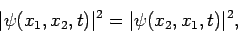(451)

or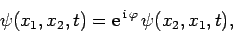(452)

where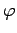is a real constant. However, if we swap the labels on particles 1 and 2 (which are, after all, arbitrary for identical particles), and repeat the argument, we also conclude that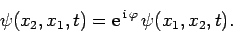(453)

Hence,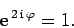(454)

The only solutions to the above equation are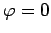and. Thus, we infer that for a system consisting of two identical particles, the wavefunction must be either symmetric or anti-symmetric under interchange of particle labels: i.e., either(455)

or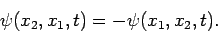(456)

The above argument can easily be extended to systems containing more than two identical particles.

It turns out that whether the wavefunction of a system containing many identical particles is symmetric or anti-symmetric under interchange of the labels on any two particles is determined by the nature of the particles themselves. Particles with wavefunctions which are symmetric under label interchange are said to obey Bose-Einstein statistics, and are called bosons--for instance, photons are bosons. Particles with wavefunctions which are anti-symmetric under label interchange are said to obey Fermi-Dirac statistics, and are called fermions--for instance, electrons, protons, and neutrons are fermions.

Consider a system containing two identical and non-interacting bosons. Let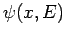be a properly normalized, single-particle, stationary wavefunction corresponding to a state of definite energy. The stationary wavefunction of the whole system is written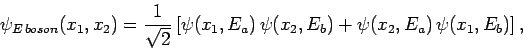(457)

when the energies of the two particles areand. This expression automatically satisfies the symmetry requirement on the wavefunction. Incidentally, since the particles are identical, we cannot be sure which particle has energy, and which has energy--only that one particle has energy, and the other.

For a system consisting of two identical and non-interacting fermions, the stationary wavefunction of the whole system takes the form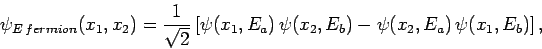(458)

Again, this expression automatically satisfies the symmetry requirement on the wavefunction. Note that if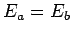then the total wavefunction becomes zero everywhere. Now, in quantum mechanics, a null wavefunction corresponds to the absence of a state. We thus conclude that it is impossible for the two fermions in our system to occupy the same single-particle stationary state.

Finally, if the two particles are somehow distinguishable then the stationary wavefunction of the system is simply(459)

Let us evaluate the variance of the distance,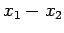, between the two particles, using the above three wavefunctions. It is easily demonstrated that if the particles are distinguishable then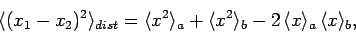(460)

where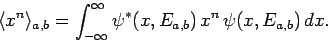(461)

For the case of two identical bosons, we find(462)

where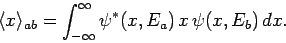(463)

Here, we have assumed that, so that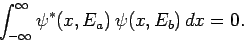(464)

Finally, for the case of two identical fermions, we obtain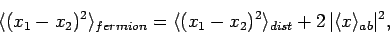(465)

Equation (462) shows that the symmetry requirement on the total wavefunction of two identical bosons forces the particles to be, on average, closer together than two similar distinguishable particles. Conversely, Eq. (465) shows that the symmetry requirement on the total wavefunction of two identical fermions forces the particles to be, on average, further apart than two similar distinguishable particles. However, the strength of this effect depends on square of the magnitude of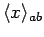, which measures the overlap between the wavefunctions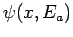and. It is evident, then, that if these two wavefunctions do not overlap to any great extent then identical bosons or fermions will act very much like distinguishable particles.

For a system containingidentical and non-interacting fermions, the anti-symmetric stationary wavefunction of the system is written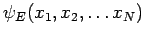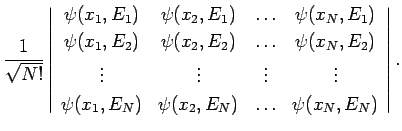(466)

This expression is known as the Slater determinant, and automatically satisfies the symmetry requirements on the wavefunction. Here, the energies of the particles are. Note, again, that if any two particles in the system have the same energy (i.e., if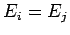for some) then the total wavefunction is null. We conclude that it is impossible for any two identical fermions in a multi-particle system to occupy the same single-particle stationary state. This important result is known as the Pauli exclusion principle.

SubsectionsNext: Exercises (N.B. Neglect spin Up: Multi-Particle Systems Previous: Two-Particle Systems
Richard Fitzpatrick 2010-07-20